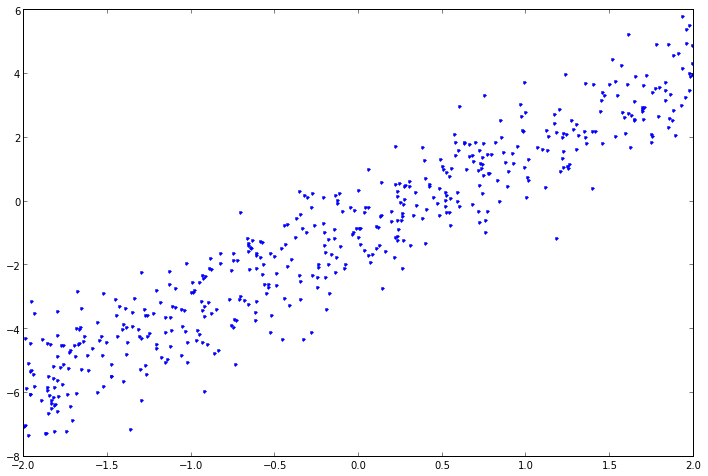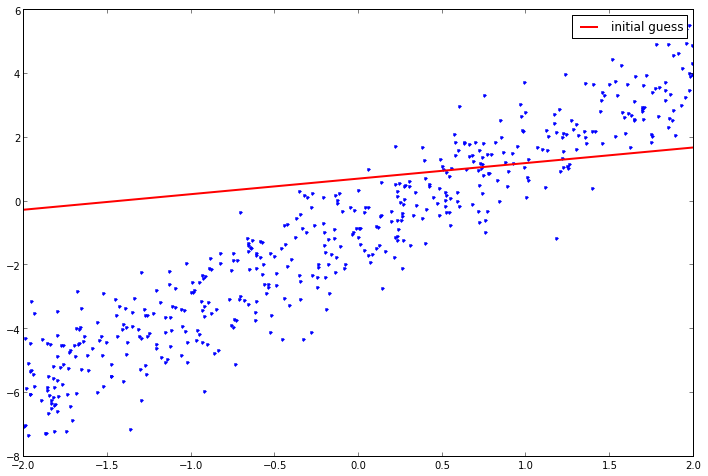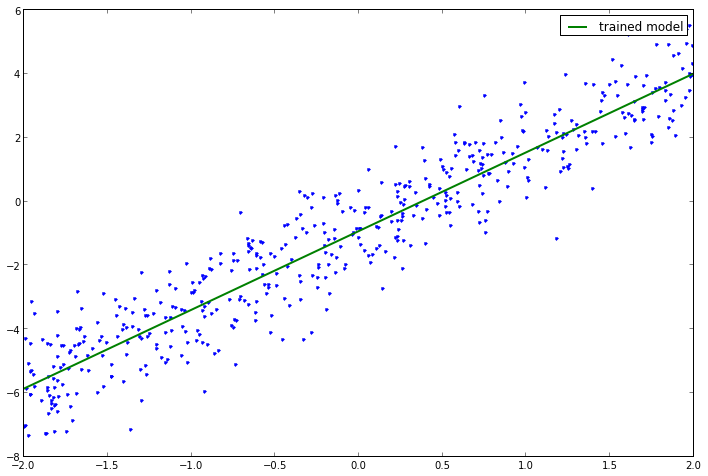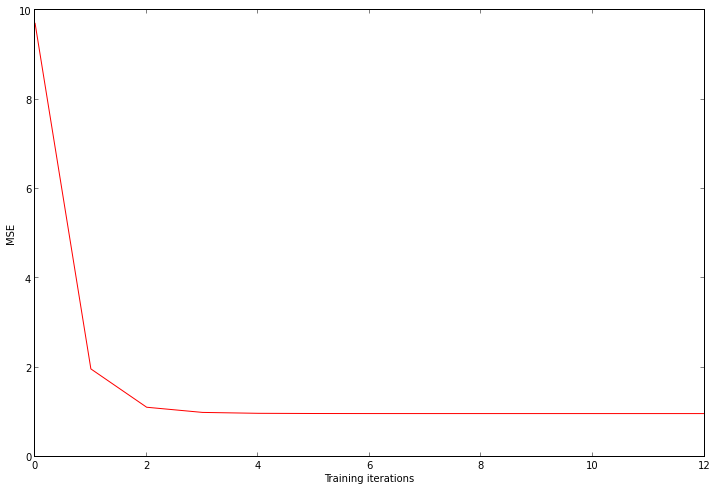# Autotelic Computing

## Saturday, October 5, 2013

### Linear Regression 101

As it looks like I found myself a new job as a data scientist (freelancing time is over!), it's time to brush up on machine learning theory and practice. Let's start with the basics: linear regression. This seems to be becoming a series of related posts, see also:
1. Linear Regression 101
2. Logistic Regression 101
3. Softmax Regression 101
4. Neural Network 101

## A Cloud of Points¶

Let's create an artificial dataset by adding some gaussian noise to points sampled along a linear function with known slope and intercept parameters. The goal will be to find (or fit) a model by allowing it to "discover" those parameters, with the help of those points only. With this model, it will be possible to predict the $y$ value of a new $x$ value, which wasn't part of the training dataset.
In :
def dataset(n, slope, intercept):
x = random.uniform(-2, 2, n)
noise = random.standard_normal([n])
y = slope * x + intercept + noise
return column_stack([x, y])

points = dataset(500, 2.5, -1)
plot(points[:,0], points[:,1], '.');## Guessing an Initial Model¶

Next we guess an initial value for $\theta$, our model parameters (with component $\theta_0$ as the intercept and $\theta_1$ as the slope). Because it is a random guess, it is initially very unlikely to be a good model of course.
In :
initial_theta = random.sample(2)
plot(points[:,0], points[:,1], '.')
plot(linspace(-2, 2), initial_theta + initial_theta * linspace(-2, 2),
'r-', label='initial guess', linewidth=2)
legend();## Training to Get Better¶

We train the parameters of the model by minimizing its error function, the mean squared error
$MSE = \frac{1}{n}\sum_{i=1}^n (h_{\theta}(x^{(i)}) - y^{(i)})^2$
where $h_{\theta}(x) = \theta_0 + \theta_1 x$, i.e. the value that the model associates to $x$, given the current state of $\theta$. The interpretation of this function is very intuitive: it's the average discrepancy between what our model says ($h$) and the training data ($y$). The better our model becomes, the lower this function should be. There are many ways to perform this minimization (including a closed-form solution which directly yields the answer, but is a little harder to derive), but here we will use a technique called batch gradient descent. The idea is to compute the derivative of the error function (or gradient) with respect to $\theta$, and iteratively take a step in the direction of steepest descent, i.e. where its value is lowered the most. The derivative of the error function is
$\frac{\partial MSE}{\partial \theta_j} = (h_{\theta}(x) - y) \cdot x_j$
Note that the error derivative is defined in terms of the components of $\theta$, of which there are two (the slope and intercept), while $x$ is a scalar. The way to treat this is to consider an implicit additional input component (or "bias") corresponding to the intercept, which has a constant value of 1. The training algorithm will iteratively update $\theta$ in proportion $\alpha$ of the gradient (to avoid taking too large steps that would make us possibly overshoot the target), and will do so in batch, meaning that it will be accumulated over the entire training set before the update occurs
$\theta_j := \theta_j - \alpha \sum_{i}^{n} (h_{\theta}(x^{(i)}) - y^{(i)}) \cdot x_j^{(i)}$
As we accumulate the MSE values while the training goes, we can stop when we find that the improvement (in terms of error reduction) between two iterations is below a certain threshold.
In :
def train(points, initial_theta, alpha=0.001, stop=1e-6):
x, y = points[:,0], points[:,1]
thetas = [initial_theta]
errors = []
while True:
theta = thetas[-1].copy()
h = theta + theta * x
theta -= alpha * sum(h - y) # intercept component
theta -= alpha * sum((h - y) * x)
thetas.append(theta)
errors.append(sum((h - y) ** 2) / len(points)) # MSE
if len(errors) > 1 and errors[-2] - errors[-1] < stop: break
return thetas, errors

thetas, errors = train(points, initial_theta)
best_theta = thetas[-1] # last one
print 'best_theta =', best_theta
plot(points[:,0], points[:,1], '.')
plot(linspace(-2, 2), best_theta + best_theta * linspace(-2, 2),
'g-', label='trained model', linewidth=2)
legend();

best_theta = [-0.91688322  2.46956016]We can see that the trained $\theta$ (slope of 2.496 and intercept of -1.04) is not so far from the original values we picked (2.5 and -1), which is a good signe. Finally, we can plot the error values, to convince ourselves that the training indeed minimized them in a consistent way.
In :
xlabel('Training iterations')
ylabel('MSE')
plot(range(len(errors)), errors, 'r-')
ylim(0);